# Standard Forms 91509 L 6 Standard Forms Copyright

• Slides: 20
Download presentationStandard Forms 9/15/09 - L 6 Standard Forms Copyright 2009 - Joanne De. Groat, ECE, OSU 1Class 6 outline o Minterms and Maxterms Sum of Products Product of Sums o Material from section 2 -3 of text o o 9/15/09 - L 6 Standard Forms Copyright 2009 - Joanne De. Groat, ECE, OSU 2Binary Function Expression o So far have seen to possible ways n n o Binary equations Truth tables What other ways are there? 9/15/09 - L 6 Standard Forms Copyright 2009 - Joanne De. Groat, ECE, OSU 3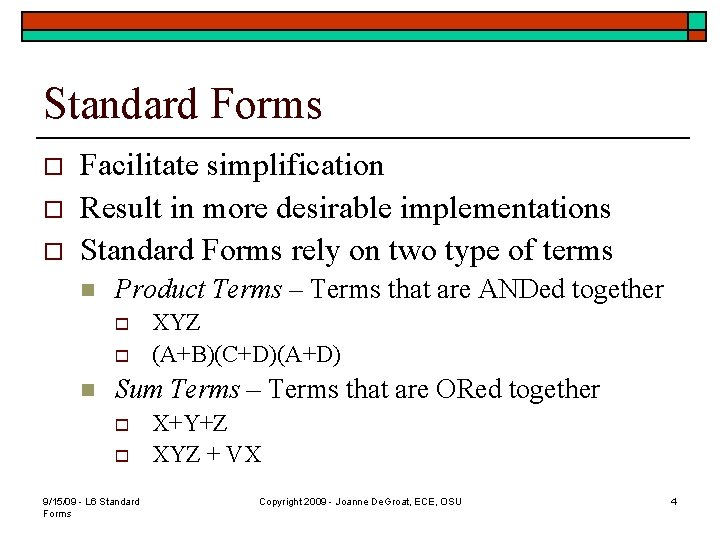Standard Forms o o o Facilitate simplification Result in more desirable implementations Standard Forms rely on two type of terms n Product Terms – Terms that are ANDed together o o n XYZ (A+B)(C+D)(A+D) Sum Terms – Terms that are ORed together o o 9/15/09 - L 6 Standard Forms X+Y+Z XYZ + VX Copyright 2009 - Joanne De. Groat, ECE, OSU 4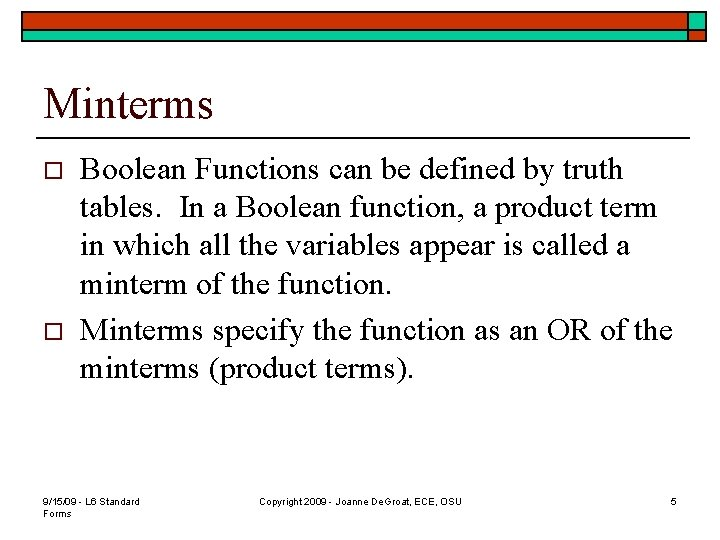Minterms o o Boolean Functions can be defined by truth tables. In a Boolean function, a product term in which all the variables appear is called a minterm of the function. Minterms specify the function as an OR of the minterms (product terms). 9/15/09 - L 6 Standard Forms Copyright 2009 - Joanne De. Groat, ECE, OSU 5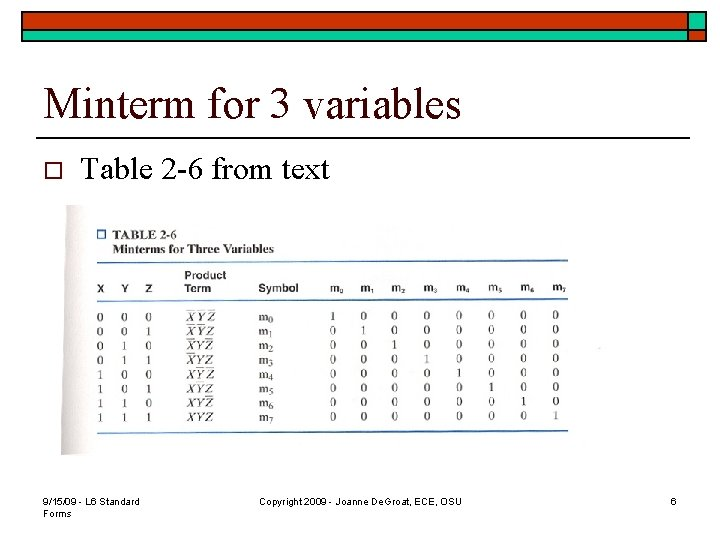Minterm for 3 variables o Table 2 -6 from text 9/15/09 - L 6 Standard Forms Copyright 2009 - Joanne De. Groat, ECE, OSU 6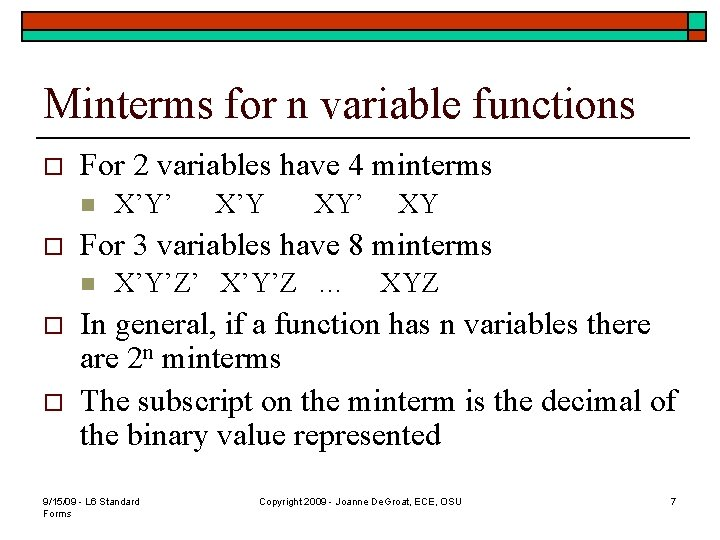Minterms for n variable functions o For 2 variables have 4 minterms n o o X’Y XY’ XY For 3 variables have 8 minterms n o X’Y’Z’ X’Y’Z … XYZ In general, if a function has n variables there are 2 n minterms The subscript on the minterm is the decimal of the binary value represented 9/15/09 - L 6 Standard Forms Copyright 2009 - Joanne De. Groat, ECE, OSU 7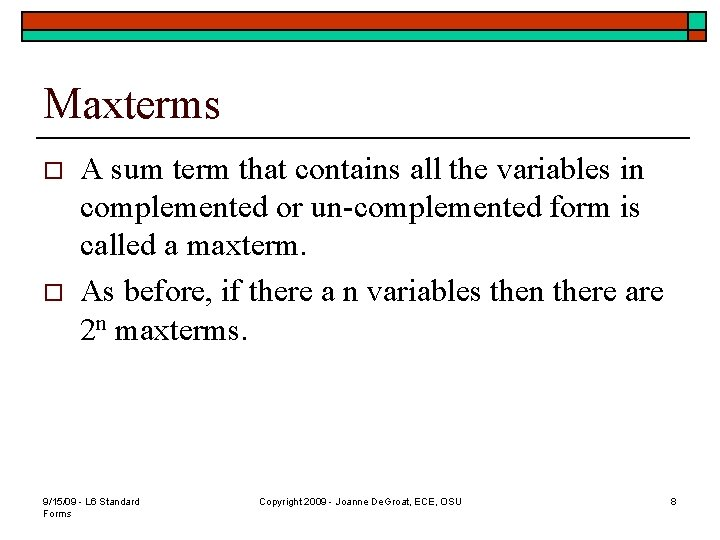Maxterms o o A sum term that contains all the variables in complemented or un-complemented form is called a maxterm. As before, if there a n variables then there are 2 n maxterms. 9/15/09 - L 6 Standard Forms Copyright 2009 - Joanne De. Groat, ECE, OSU 8Maxterm table o The maxterms 9/15/09 - L 6 Standard Forms Copyright 2009 - Joanne De. Groat, ECE, OSU 9Specifying functions o o Functions can be specified in minterm or maxterm notation Minterm n n n F(X, Y, Z) = ∑m(0, 2, 5, 7) = X’Y’Z’ + X’YZ’ + XY’Z + XYZ And then you can work on simplifying this Or could have also had F(X, Y, Z) = m 0 + m 2 + m 5 + m 7 9/15/09 - L 6 Standard Forms Copyright 2009 - Joanne De. Groat, ECE, OSU 10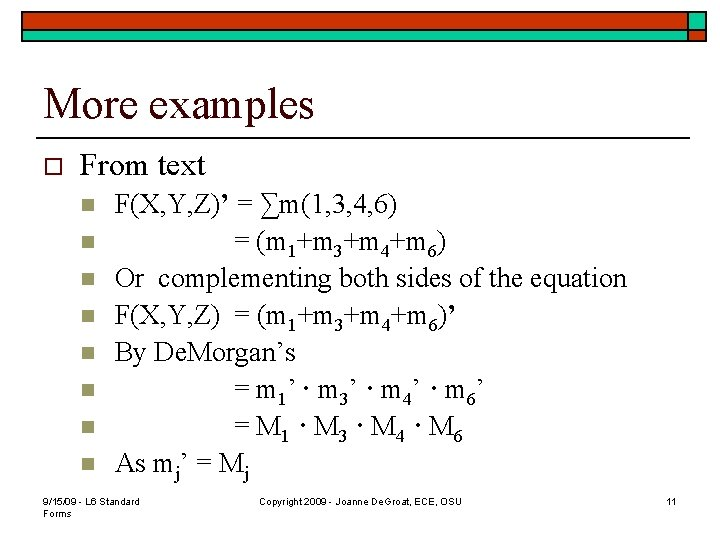More examples o From text n n n n F(X, Y, Z)’ = ∑m(1, 3, 4, 6) = (m 1+m 3+m 4+m 6) Or complementing both sides of the equation F(X, Y, Z) = (m 1+m 3+m 4+m 6)’ By De. Morgan’s = m 1’ · m 3’ · m 4’ · m 6’ = M 1 · M 3 · M 4 · M 6 As mj’ = Mj 9/15/09 - L 6 Standard Forms Copyright 2009 - Joanne De. Groat, ECE, OSU 11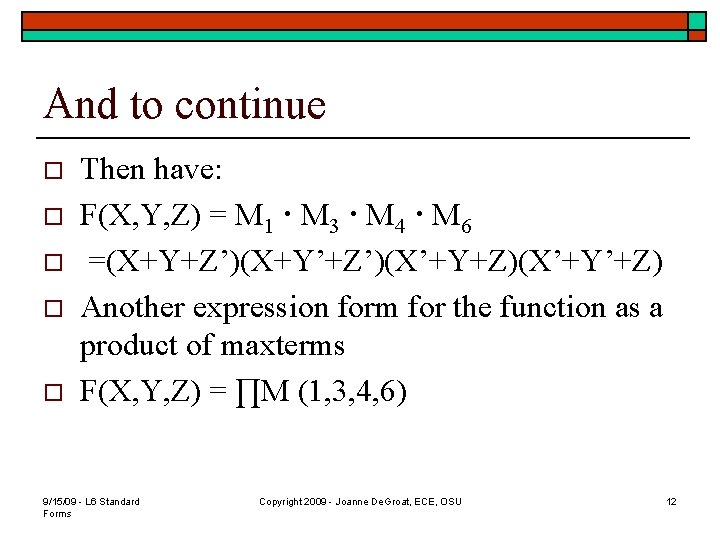And to continue o o o Then have: F(X, Y, Z) = M 1 · M 3 · M 4 · M 6 =(X+Y+Z’)(X+Y’+Z’)(X’+Y+Z)(X’+Y’+Z) Another expression form for the function as a product of maxterms F(X, Y, Z) = ∏M (1, 3, 4, 6) 9/15/09 - L 6 Standard Forms Copyright 2009 - Joanne De. Groat, ECE, OSU 12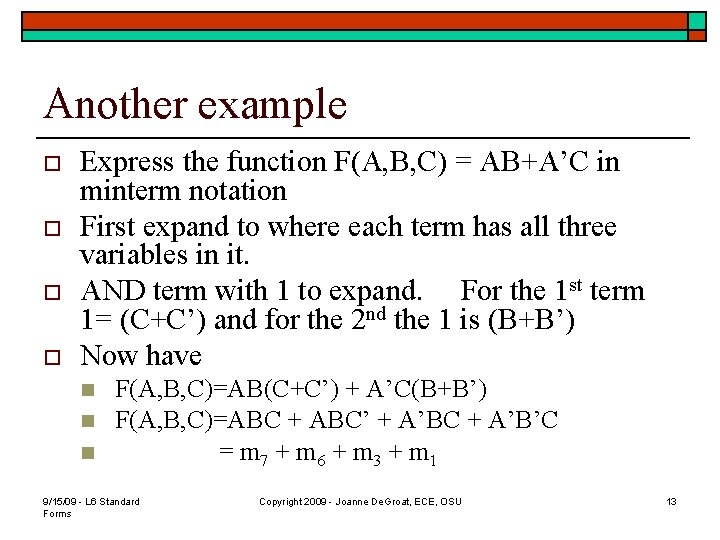Another example o o Express the function F(A, B, C) = AB+A’C in minterm notation First expand to where each term has all three variables in it. AND term with 1 to expand. For the 1 st term 1= (C+C’) and for the 2 nd the 1 is (B+B’) Now have n n n F(A, B, C)=AB(C+C’) + A’C(B+B’) F(A, B, C)=ABC + ABC’ + A’BC + A’B’C = m 7 + m 6 + m 3 + m 1 9/15/09 - L 6 Standard Forms Copyright 2009 - Joanne De. Groat, ECE, OSU 13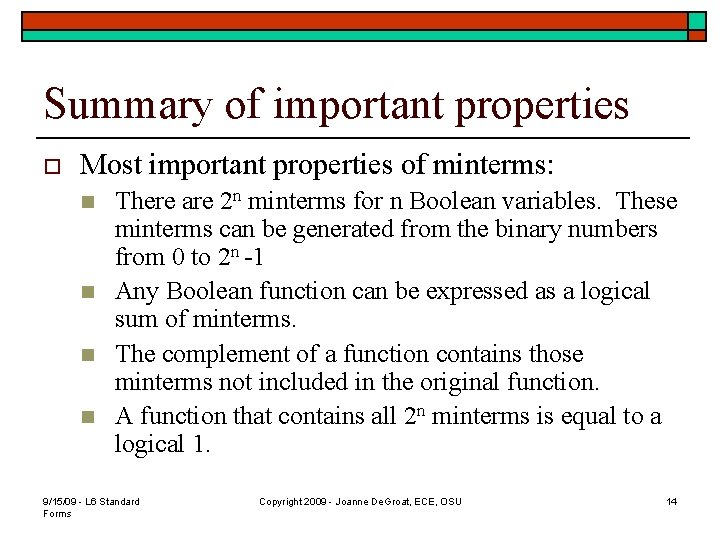Summary of important properties o Most important properties of minterms: n n There are 2 n minterms for n Boolean variables. These minterms can be generated from the binary numbers from 0 to 2 n -1 Any Boolean function can be expressed as a logical sum of minterms. The complement of a function contains those minterms not included in the original function. A function that contains all 2 n minterms is equal to a logical 1. 9/15/09 - L 6 Standard Forms Copyright 2009 - Joanne De. Groat, ECE, OSU 14Expansion of another function o Express E = Y’ + X’Z’ in minterm notation. n n n o E = (X+X’)Y’ + X’Z’(Y+Y’) = XY’(Z+Z’) + X’Y’(Z+Z’)+X’YZ’+X’Y’Z’ = XY’Z+XY’Z’+X’Y’Z’+X’YZ’ = m 5 + m 4 + m 1 + m 0 + m 2 = m 5 + m 4 + m 2 + m 1 + m 0 Text shows how to find the minterm expression using a truth table. 9/15/09 - L 6 Standard Forms Copyright 2009 - Joanne De. Groat, ECE, OSU 15Using a truth table o E = Y’ + X’Z’ Have those entries that are Y’ o Those that are X’Z’ o giving o 9/15/09 - L 6 Standard Forms Copyright 2009 - Joanne De. Groat, ECE, OSU 16Sum-of-Products o Starting with the minterm specification of a function n o o o F(X, Y, Z) = ∑m(2, 3, 4, 7) = (m 2+m 3+m 4+m 7) = X’YZ’ + X’YZ + XY’Z’ + XYZ Each minterm represents a product term and then we sum them to generate the function. This form is called sum-of-products. Even when in minimal form it is still the sum-ofproducts. 9/15/09 - L 6 Standard Forms Copyright 2009 - Joanne De. Groat, ECE, OSU 17Producing sum-of-products o Form other form of the function n n F = AB + C(D + E) Can distribute the C F = AB + CD + CE And now have the function in sum-of-products form. The sum-of-products form is a 2 level implementation of the function in gates 9/15/09 - L 6 Standard Forms Copyright 2009 - Joanne De. Groat, ECE, OSU 18Product-of-sums o o What the local grocer does? This form arises from the Maxterm representation of a function. n n o o F(X, Y, Z) = ∏M (2, 3, 4, 6) = (X+Y’+Z)(X+Y’+Z’)(X’+Y+Z)(X’+Y’+Z) And now have F in a product-of-sums form Once again, a 2 level circuit. 9/15/09 - L 6 Standard Forms Copyright 2009 - Joanne De. Groat, ECE, OSU 19Class 6 assignment o o Covered section 2 -3 Problems for hand in n o 2 -13 Problems for practice n o 2 -11 2 -10 Reading for next class: section 2 -4 9/15/09 - L 6 Standard Forms Copyright 2009 - Joanne De. Groat, ECE, OSU 20